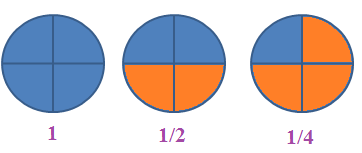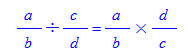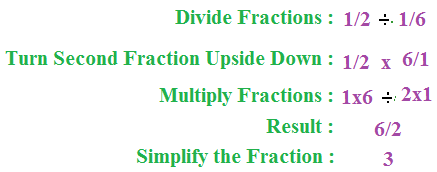## What is a fraction

A fraction is a number we need for measuring. It is actually a part of a whole or, more generally, any number of equal parts. But a mathematical fraction is not simply a part of anything. It is a part of the unit of measure, which is 1.Eg: 1/4

1/4 means 1 out of 4

### Numerator / Denominator

We call the top number the Numerator, it is the number of parts you have. Here in the above case 1 is Numerator, we call the bottom number the Denominator, it is the number of parts the whole is divided into. Here in the above case 2 is the Denominator.

### How to divide fractions

Dividing fractions can be a little tricky, that is multiply the number by the reciprocal of the fraction.

The algebraic formula for dividing fractions is :For example, the reciprocal of 2/4 is 4/2.

### Dividing Fractions#### VB.Net Implementation

```Public Class Form1
Private Sub Button1_Click(ByVal sender As System.Object, ByVal e As System.EventArgs) Handles Button1.Click
Dim result1 As Double
Dim result2 As Double
result1 = ((1 / 2) / (1 / 6))
result2 = ((1 / 2) * (6 / 1))
MsgBox(result2)
End Sub
End Class
```

#### C# Implementation

```using System;
using System.Collections.Generic;
using System.ComponentModel;
using System.Data;
using System.Drawing;
using System.Linq;
using System.Text;
using System.Windows.Forms;
namespace WindowsFormsApplication1
{
public partial class Form1 : Form
{
public Form1()
{
InitializeComponent();
}
private void button1_Click(object sender, EventArgs e)
{
double  result1 = ((1.0 / 2.0) / (1.0 / 6.0)) ;
double result2 = ((1.0 / 2.0) * (6.0 / 1.0));
MessageBox.Show(result1.ToString());
}
}
}
```